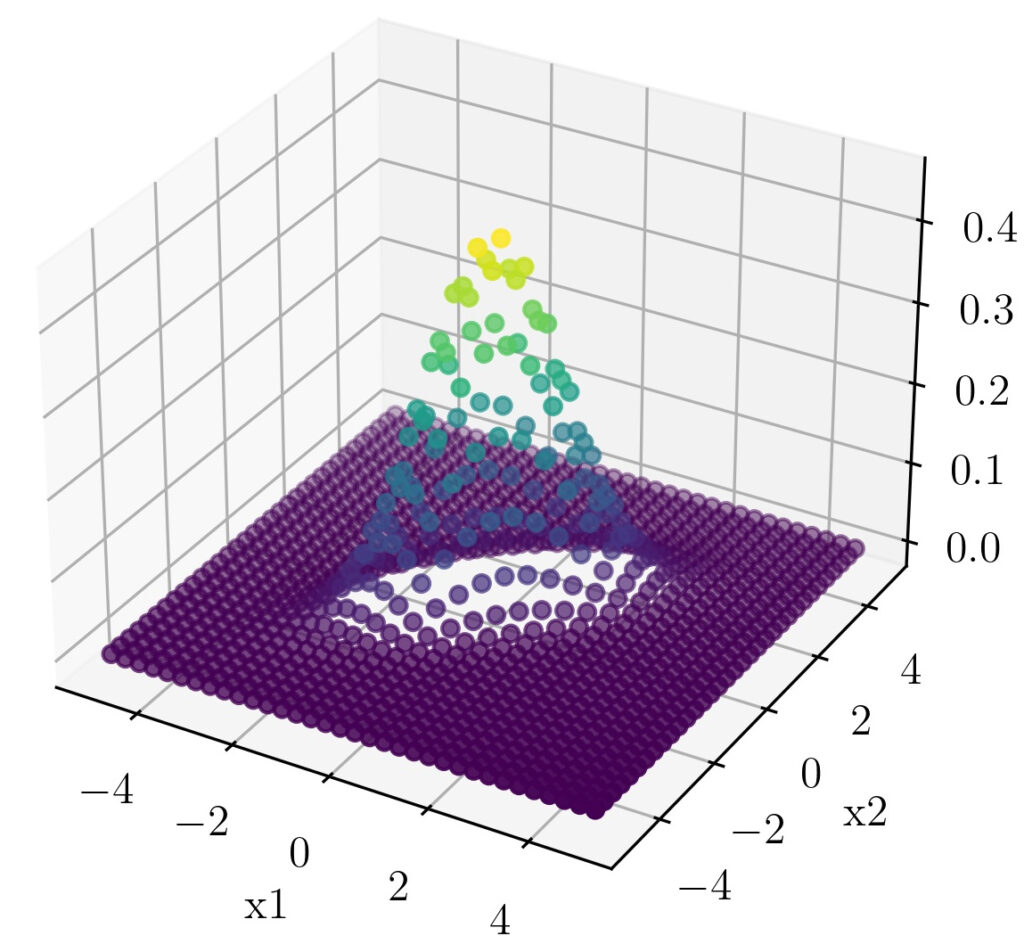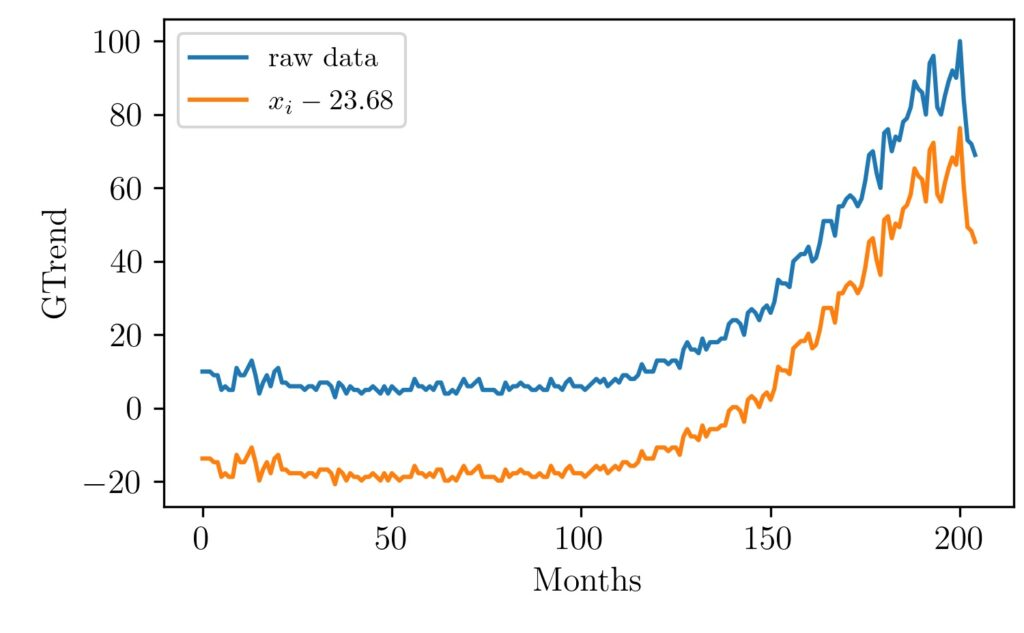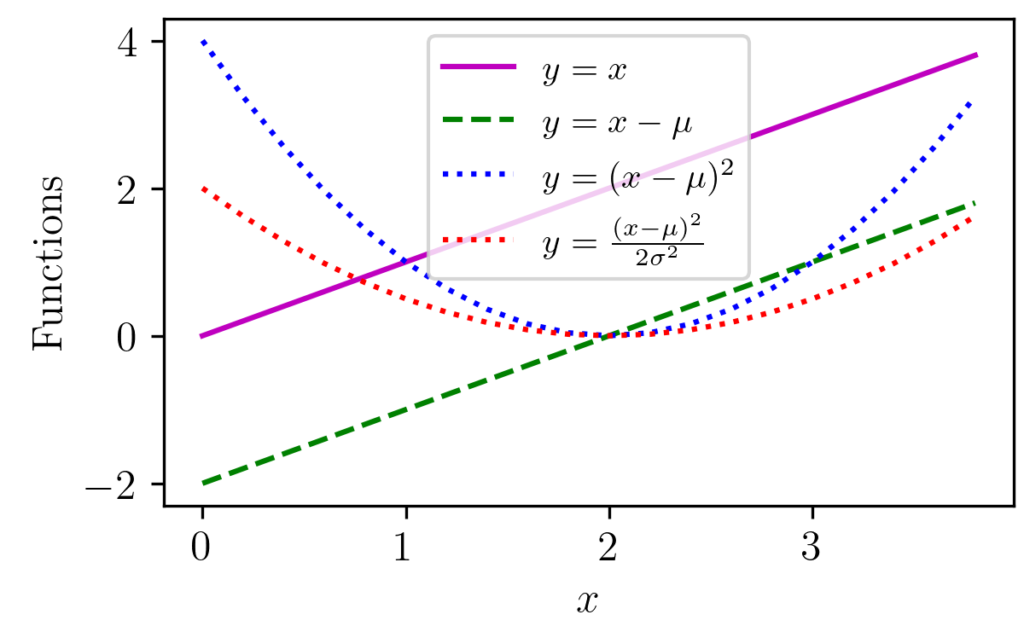# Sajil C. K.

I am an inquisitive person with interests falling at the intersection of mathematics, programming, and research. I like to understand concepts to the core by building things from scratch.## Mathematical maturity

The topic I wish to talk in this post is about Mathematical maturity. It is connected to the abstract nature of mathematics and humans’ endeavor to cope with it. Let me start by asking you a question. Have you ever seen a circle in real-life?. You might say yes because we all draw many in …

## Review of RL Tutorial from Center for Brains Minds and Machines

The Center for Brains, Minds and Machines (CBMM) is a premier institute and NSF Science and Technology Center dedicated to the study of intelligence. The website and Youtube channel of the institute contains a good number of tutorials and educational materials that help anyone interested in topics related to neuroscience, machine intelligence, etc. This tutorial …

Introduction The field of machine learning and artificial intelligence is very promising. The scientific progress and interest in the field are increasing every day. The applicability of the ideas into multiple fields makes it attractive to people from a diverse skill set. Subject experts in this field are often transitioned from other technical domains like …

## The Art of Dividing and Conquering

Introduction Divide and conquer seem the most widely used strategy to attack hard problems. The use of this idea and similar approaches are ample in every discipline. In this post, let’s look at how this concept is a cornerstone in analyzing signals and systems. Consider the case of audio signals. We can think of them …## Hacking the Bivariate Gaussian Distribution

In one of our earlier posts, we have seen how we can visually relate the parts of the one-dimensional Gaussian distribution equation. In this post, we will follow the same strategy to understand the terms that comes up with a Multivariable Gaussian distribution. We will focus on the Bivariate Gaussian distribution as distributions of higher-order …## Visualizing MinMax Scaling

Normalization of vectors, an array of values, signals is often used as a preprocessing step before many algorithms. For example, in machine learning, some types of algorithms are prone to different inherent scales of features. In such situations normalization is done to give the same weightage to all features before passing it to the algorithm …## Gaussian Distribution Explained Visually

Gaussian distribution appears in various parts of science and engineering. Apart from a distribution often appear in nature, it has got important properties such as its relation to Central Limit Theorem (CLT). The figure above shows one-dimensional Gaussian distributions of various mean and variance values. Libraries like NumPy provide functions that can return Gaussian distribution …## Reinforcement Learning Resources

Following is a list (in progress) of resources on Reinforcement Learning and allied topics. Though not comprehensive, it includes University lectures, YouTube playlists, MOOCs, blogs, etc. Hope this would be useful to someone getting started in reinforcement learning. UC Berkely CS 285 at UC Berkeley Deep Reinforcement Learning (23) Deep RL Bootcamp (15) CS 294: …## What does it mean to say orthogonal?

In this article, let us explore what is meant by the concept of orthogonality. Orthogonal or orthogonality is often introduced from a mathematical perspective. In general, most resources explains it in terms of linear algebra and vectors. One such example is when we learn about right-angled triangles the two sides are drawn as perpendicular or …